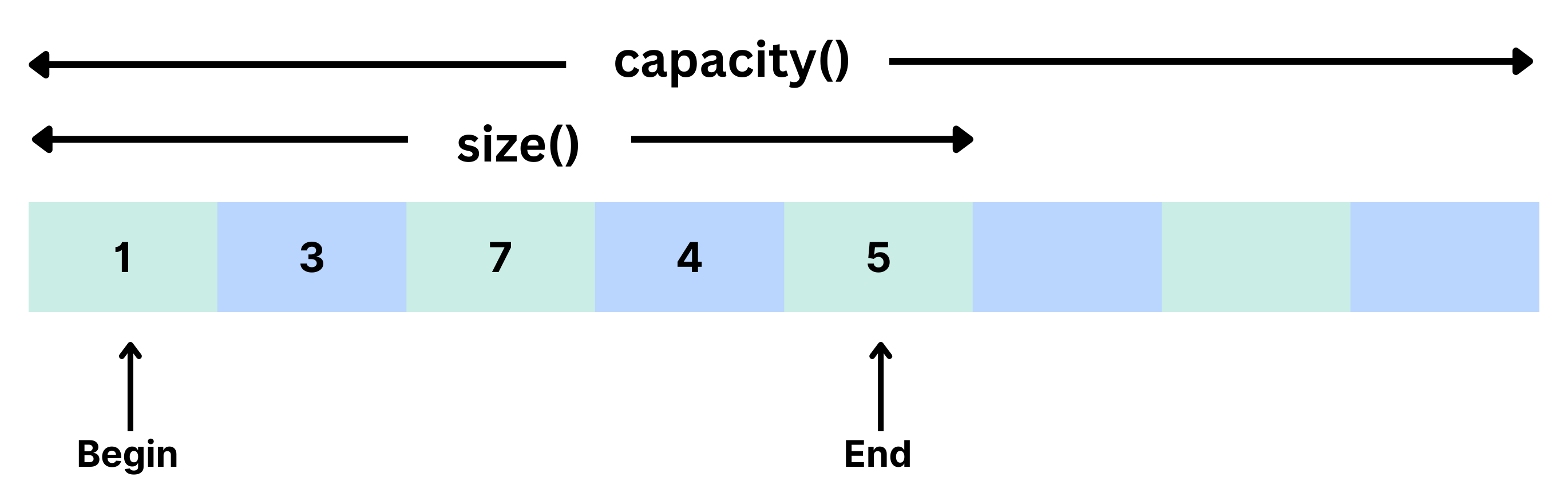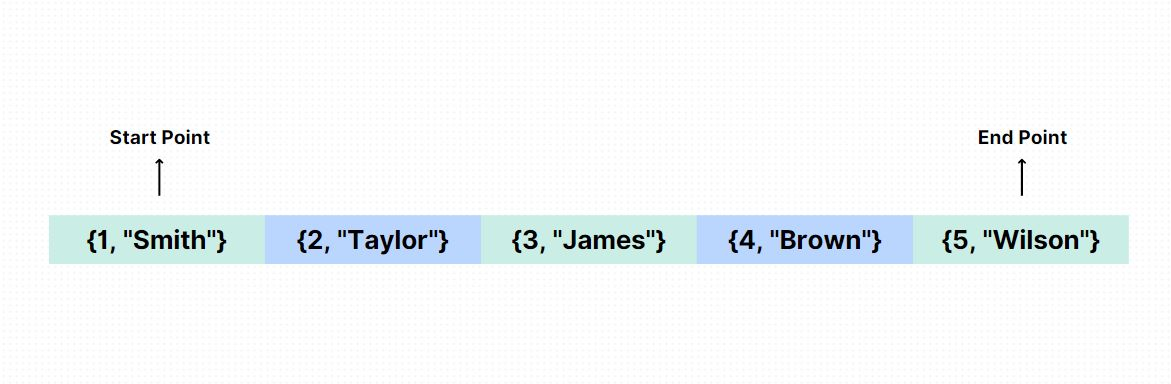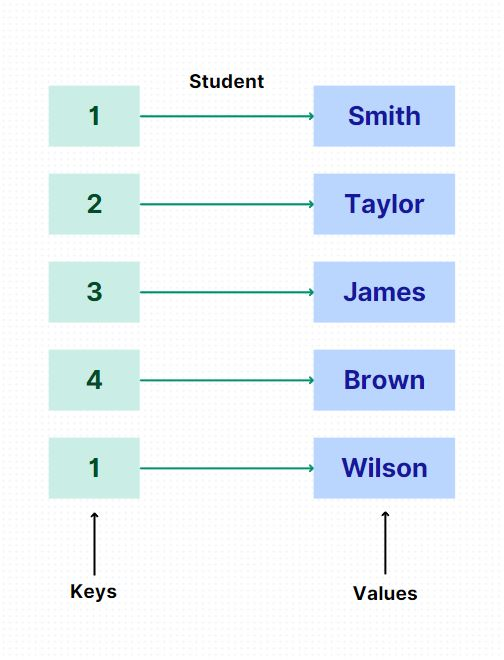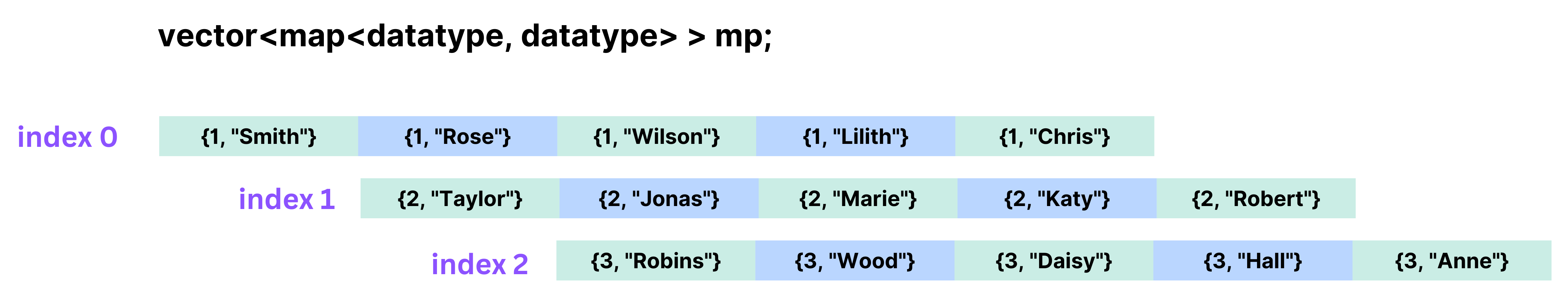×

Search anything:

# Vector of Map in C++

#### C++Get this book -> Problems on Array: For Interviews and Competitive Programming

• What is Vector
• What is Map
• What is Vector of Map
• Complexity
• Some questions

# What is Vector?

In this article at OpenGenus, we will mainly know about vector of map. Beforehand, we will know briefly about what is vector in C++. Vector is a part of Standard Template Library of C++. It is mostly like array but the key difference is the size of the vector can be defined or changed dynamically which means during the execution of our program.

Here is the visualization:We can declare a vector like this:

``````vector <data type> vector_name;
``````

We can add items in vector dynamically by this:

``````vector.push_back(item);
``````

Also items can be removed dynamially using this:

``````vector.pop_back(item);
``````

Items are always appended at the end and removed from the end of the vector.

# What is Map

Map is a STL container that is usually used for storing paired data. The paired data are called key-value pairs. Normally, one key can not have more than one value in map.
For better understanding:The key-value pair can be visualized this way:Map can be declared like this:

``````map<data type, data type> map_name;
``````

if we want to insert some pairs into map then:

``````map_name [key] = value;
``````

There are two types of map: ordered map and unordered map. When the map is declared normally then it is declared as ordered map. We can declare unordered map like this:

``````unordered_map<data type, data type> map_name;
``````

# What is Vector of Map

Now it's easier to comprehend what vector of map is. It is collection of map stored in vector. It is useful to design efficiten data structure. We can see here:We can declare it like this:

``````vector<map<data type, data type>> vector_name;
``````

We create maps and then add it to vector like this:

``````vector<map<data type, data type>> vector_name;
map<data type, data type> map_name;
vector_name.push_back(map_name);
``````

Here is a full code on how we should work on it:

``````#include <iostream>
#include <vector>
#include <map>

int main() {
std::vector<std::map<std::string, int>> students; // Create a vector of maps

// Create a map for the first student
std::map<std::string, int> student1;
student1["id"] = 1;
student1["age"] = 18;

// Add the first student to the vector
students.push_back(student1);

// Create a map for the second student
std::map<std::string, int> student2;
student2["id"] = 2;
student2["age"] = 19;

// Add the second student to the vector
students.push_back(student2);

// Access elements in the vector of maps
std::cout << "First student ID: " << students["id"] << std::endl;

return 0;
}
``````

What is the possible outcome of the code?

• 1, 85
• 1, 18
• 1, 90
• 1, 19

The answer is 1, 90. The students indicates student1 map and students indicates student2 map.

In the above example, we created a vector called students, which holds maps with std::string keys and int values. We created individual maps for each student and populate them with key-value pairs using the [] operator. The maps represent different attributes of the students, such as ID, age, and grade. We can add more attributes as we need.
We then added the maps to the vector using the push_back function. To access elements in the vector of maps, we used the indexing operator [] to access a particular map, and then use the [] operator again on the map to retrieve the value associated with a specific key.
We can add more key-value pairs to each map, modify the values, or iterate over the vector of maps using loops like range-based for loops or iterators.
We should remember that when working with maps, the keys must be unique within each map. If we attempt to insert a key that already exists, it will update the corresponding value rather than creating a new entry.

We can also redo the code using unordered map. In that case the advantage would be the access time to elements is constant on average, regardless of the size of the map. However, the order of elements is not guaranteed in an unordered map.

It's important to note that the actual performance may vary based on the specific implementation and the hashing function used by the unordered map container.

# Complexity

The complexity of inserting an element into a vector of maps in C++ depends on two factors: the complexity of inserting an element into a map, and the complexity of inserting an element into a vector.

1. Inserting an element into a map:
• The average case complexity for inserting an element into a map is logarithmic O(log n), where 'n' is the number of elements already in the map.

• The worst-case complexity for inserting an element into a map is linear O(n), which occurs when there are many collisions and the map needs to rehash its internal structure.
Inserting an element into a vector:

1. The complexity of inserting an element into a vector depends on where the element is inserted:
• If the element is inserted at the end using the push_back function, the average and amortized complexity is constant O(1). This is because vectors allocate extra memory and resizing is done infrequently.
• If the element is inserted at a specific position using the insert function, the complexity is linear O(n), as all elements after the insertion point need to be shifted to accommodate the new element.

Considering these factors, inserting an element into a vector of maps involves inserting the element into the map (logarithmic complexity) and then inserting the map into the vector (constant or linear complexity). Therefore, the overall complexity of inserting an element into a vector of maps can be summarized as follows:

• Average case: O(log n) + O(1) = O(log n)
• Worst case: O(log n) + O(n) = O(n)

It's important to note that these complexities assume that the keys in the maps are properly distributed to minimize collisions. If the keys are not distributed well, the worst-case complexity for inserting into the maps could be higher.

Also, in case of unordered map, The complexity of inserting an element into an unordered map is constant on average O(1), while the complexity of inserting an element into a vector is constant or linear, depending on the insertion position. Therefore, the overall complexity of inserting an element into a vector of unordered maps is the same as that of inserting into an unordered map, which is O(1) on average.

# Questions

1. What is the average case complexity of inserting an element into a vector of maps?
• O(n)
• O(logn)
• O(1)
• O(nlogn)

2. What is the average case complexity of inserting an element into a vector of unordered maps?

• O(n)
• O(logn)
• O(1)
• O(nlogn)

3. How do we declare vector of map?

``````map<vector<string> vector_name, string>map_name;
``````
``````vector<map<string,string>> vector_name;
``````
``````vector<map<string,string>,map<int,int>> vector_name;
``````

or

``````map<vector<string> vector_name, vector<char> vector_name>map_name;
``````

``````vector<map<string,string>> vector_name;